282
Created byMaster Student

Chemistry Practice Test #3

1 / 30

The simplest alkene has

2 / 30

A certain reaction has a ΔH° = -43.2 kJ mol-1 and an entropy change ΔS° of +22.0 J mol-1 K-1. What is the value of ΔG° at 800 °C?

3 / 30

The oxidation number of chlorine in the perchlorate ion, ClO4, is

4 / 30

What is the equilibrium expression for the reaction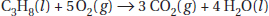• A.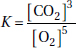• B.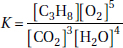• C.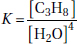• D.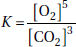5 / 30

Which of the following is a conjugate acid-base pair?

6 / 30

How many electrons, neutrons, and protons are in an atom of 52Cr?

7 / 30

Which of the following can form hydrogen bonds?

8 / 30

Which of the following reactions is expected to have the greatest decrease in entropy?

9 / 30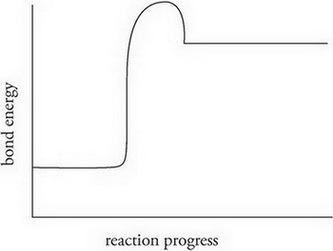The concentrations of the reactants and products in the reaction represented by the above graph are found to be changing very slowly. Which of the following statements best describes the reaction given that the reaction is exergonic? (ΔG < 0)

10 / 30

The ideal gas law is successful for most gases because

11 / 30

What is the work involved if a gas in a 2.0-litre container at 2.4 atmospheres pressure is allowed to expand against a pressure of 0.80 atmospheres?

12 / 30

All of the following ions have the same electron configuration except

13 / 30

The subatomic particle with the most penetrating ability is

14 / 30

Helium effuses through a pinhole 5.33 times faster than an unknown gas. That gas is most likely

15 / 30

NO2 + O3 → NO3 + O2 slow
NO3 + NO2 → N2O5 fast

A proposed reaction mechanism for the reaction of nitrogen dioxide and ozone is detailed above. Which of the following is the rate law for the reaction?

16 / 30

A first-order reaction has a half-life of 34 minutes. What is the rate constant for this reaction?

17 / 30

The electron configuration 1s2 2s2 2p6 3s2 3p6 4s2 signifies the ground state of the element

18 / 30

The following diagram supports which of the following conclusions about the reaction shown below?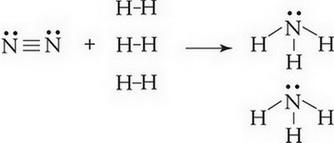19 / 30

A mechanism is a sequence of elementary reactions that add up to the overall reaction stoichiometry. A substance that is produced in one elementary reaction and consumed in another is called

20 / 30

Changing which of the following will change the numerical value of the equilibrium constant?

21 / 30

Solvents A and B form an ideal solution. Solvent A has a vapour pressure of 345 torrs at room temperature. At the same temperature, solvent B has a vapour pressure of 213 torrs. What is the vapour pressure of a mixture where the mole fraction of A is 0.285?

22 / 30

Which of the following is expected to be a polar molecule?

23 / 30

A solution is prepared by dissolving 30.0 grams of Ni(NO3)2 in enough water to make 250 mL of solution. What is the molarity of this solution?

24 / 30

What is the molarity of a sodium hydroxide solution that requires 42.6 mL of 0.108 M HCl to neutralize 40.0 mL of the base?

25 / 30

Which of the following correctly lists the individual intermolecular attractive forces from the strongest to the weakest?

• A. Induced dipole < dipole-dipole < hydrogen bond
• B. Hydrogen bond < dipole-dipole < induced dipole
• C. Induced dipole < hydrogen bond < dipole-dipole
• D. Dipole-dipole < hydrogen bond < induced dipole

26 / 30

A certain radioactive material has a half-life of 36 minutes. Starting with 10.00 grams of this material, how many grams will be left after 2 hours?

27 / 30

The equilibrium constant, Kc, for the dissociation of HI into hydrogen gas and iodine vapour is 21 at a certain temperature. What will be the molar concentration of iodine vapour if 15 grams of HI gas is introduced into a 12.0-L flask and allowed to come to equilibrium?

28 / 30

How many moles of propane (C3H8) are there in 6.2 g of propane?

29 / 30

What is the molar mass of a monoprotic weak acid that requires 26.3 mL of 0.122 M KOH to neutralize 0.682 gram of the acid?

30 / 30

The Pauli exclusion principle states that